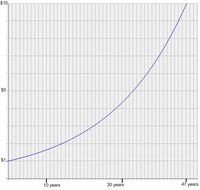#Difference Between Similar Terms and Objects

# Difference Between Simple and Compound InterestSimple Interest vs Compound Interest

Interest rate is generally defined as the cost for borrowing money. It is stated in percentage and set against the original amount of the borrowed money or the principal. There are two types of interests. One is simple interest while the other is compound interest. If you are planning to borrow money or to invest in the money market, you should get a clear idea about the difference between simple and compound interest.

First of all, simple interest is computed based on the principal only or original amount of the borrowed money. Compound interest on the other hand is computed periodically. The computations include the earned interest from the principal plus the compounded interest earned over a period of time.

Simple interest is applied on loans for single periods such as 30 days or 60 days. So if you get a short term loan for 60 days, the interest will be computed based on the original principal only. For long term loans, lenders usually apply compound interest. The periods are pre-defined by the lender. These could be quarterly, semi-annually, or annually. With compound interest, the interest earned during the previous period will be added to the principal. This will become the new principal and it will earn an interest according to the terms agreed.

You have to note that the growth of interest with simple interest calculation is constant. That is why it is the preferred system for short-term loans. With compound interest, the growth is exponential because the principal is getting bigger each period. Compounding interest is the fastest way to accumulate wealth so this is the preferred system of lenders and investors.

Simple interest and compound interest are radically different from each other. The former is usually applied to short-term loans while the latter is used for long term loans and investments.

Latest posts by Mabelle (see all)

### Search DifferenceBetween.net :

Custom Search

Help us improve. Rate this post!(12 votes, average: 4.42 out of 5)Loading...Email This Post : If you like this article or our site. Please spread the word. Share it with your friends/family.

### 1 Comment